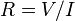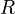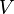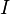# Ohm facts for kids

Kids Encyclopedia Facts

There are some materials which offer some resistance to the current flow, they are called resistive materials, for example graphite, iron, and certain synthetic materials.

The magnitude that represents this characteristic is the resistance, and it is measured in ohms. An ohm (referred to by the Greek letter Ω (Omega)) is the SI unit of resistance (R). It can be defined by Ohm's law and the equation:$R= V/I$

where${\textstyle R}$ is resistance in ohms,${\textstyle V}$ is voltage in volts, and${\textstyle I}$ the current in amperes.

The ohm was named after Georg Ohm.Ohm Facts for Kids. Kiddle Encyclopedia.